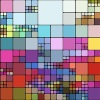#### You may also like### Sine Problem

In this 'mesh' of sine graphs, one of the graphs is the graph of the sine function. Find the equations of the other graphs to reproduce the pattern.### Squaring the Rectangle

Can you find a way to turn a rectangle into a square?### The Use of Mathematics in Computer Games

An account of how mathematics is used in computer games including geometry, vectors, transformations, 3D graphics, graph theory and simulations.

# Footprints

##### Age 16 to 18Challenge Level

Investige combinations of two reflections in parallel and in intersecting mirror lines.

Suppose you have an infinite frieze pattern of footsteps. Investigate a combination of reflections that will give a glide reflection mapping any footstep in the pattern to the next one. Where would the mirror lines have to be? Show that, with the mirror lines you have chosen, the third step maps to the fourth, the fourth to the fifth and so on...